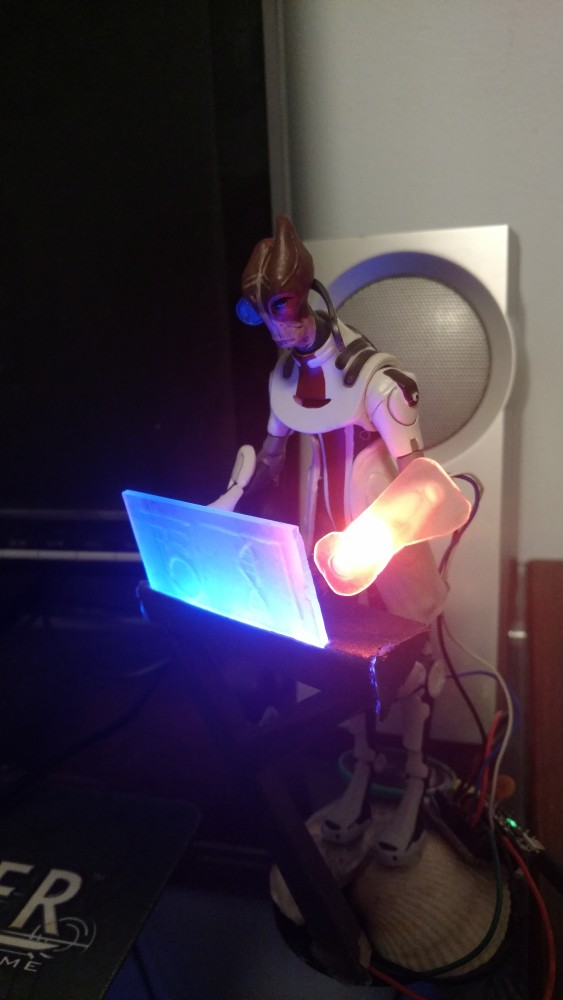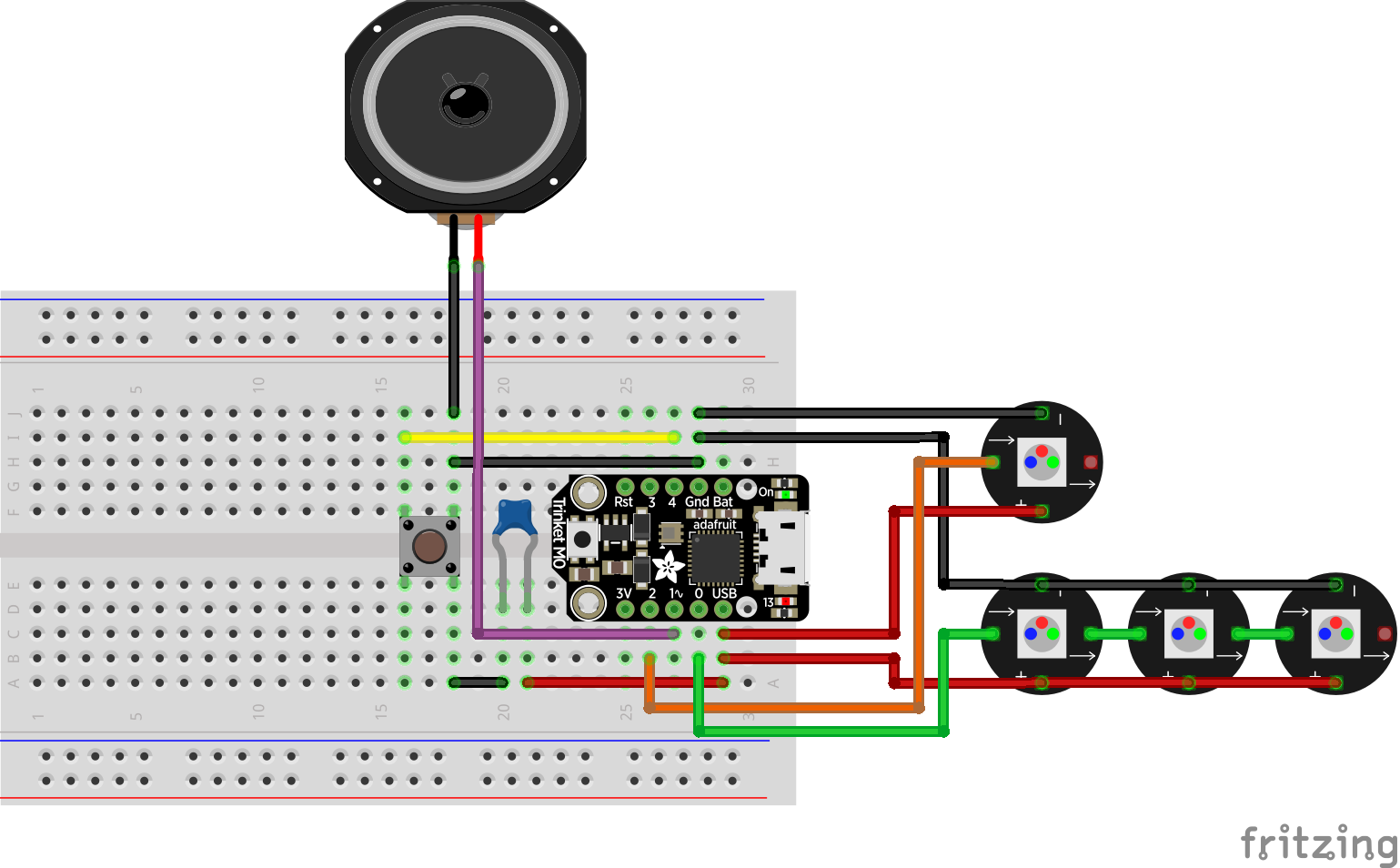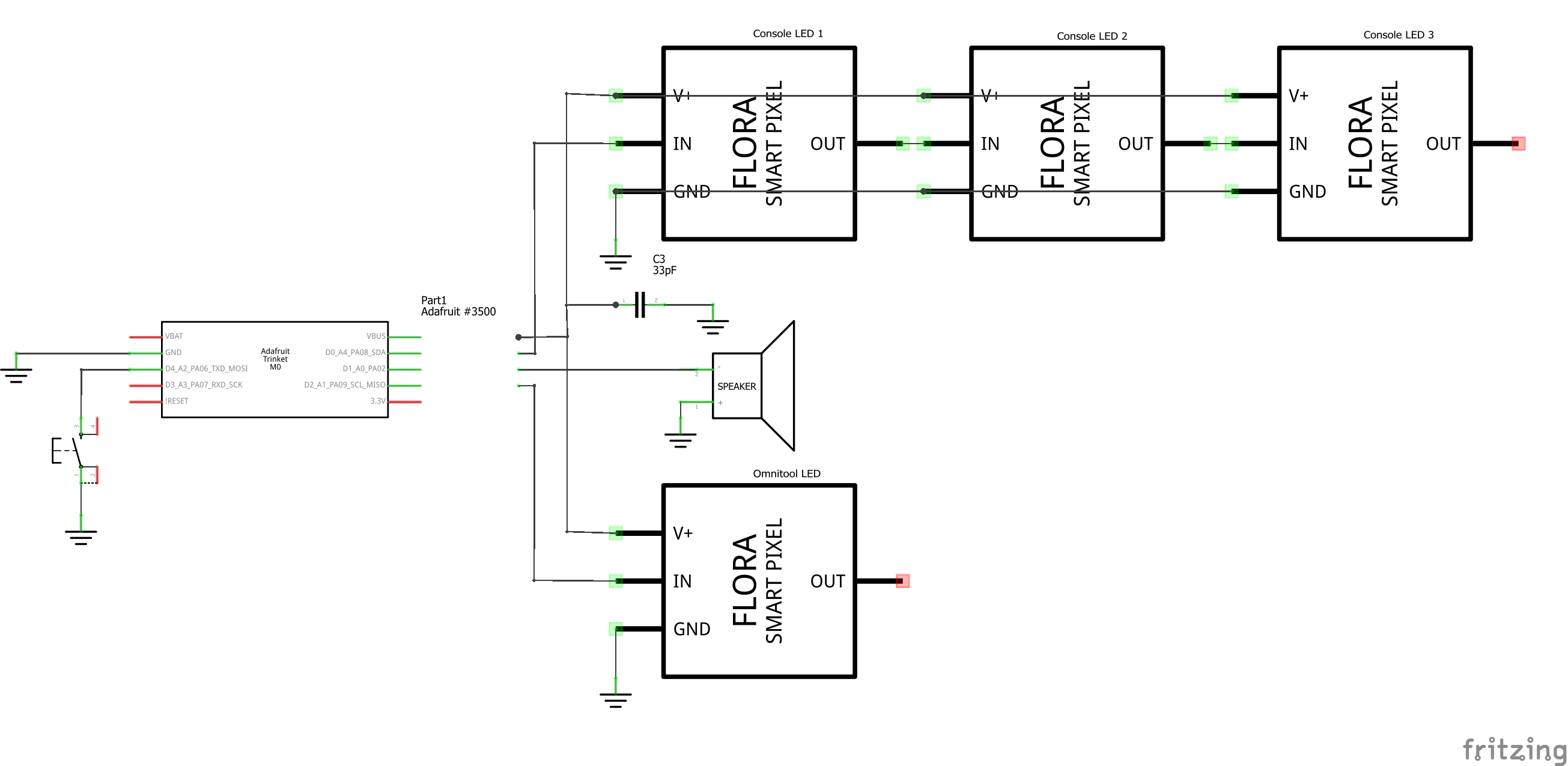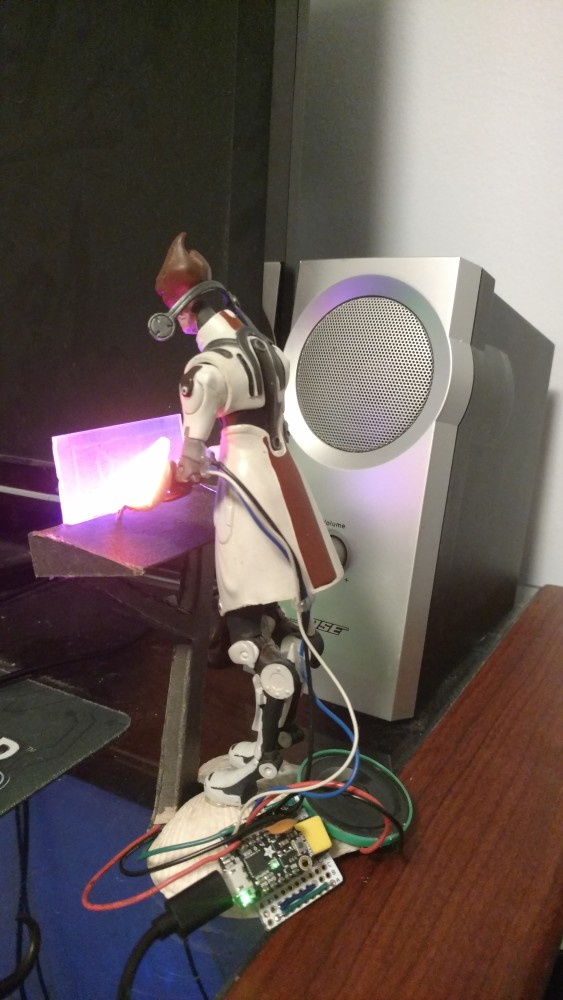# Mordin Solus

Published: Apr 29, 2020 by K. E. Claytor

# Mordin Solus Timer

Kiddo found an old Mordin Solus (Mass Effect) action figure I had and wanted to know when it would light up again. So we added four RGB LEDs, a speaker, and a button and turned it into a ambient light and pomodoro timer.

Here’s the summary video:

This project also appears at:

This project costs at most \$21 (cheaper if you have some parts already) and is easy:

• Hardware: Easy
• Software: Easy
• Assembly: Easy# Hardware

The electronics parts list consist of:

• Trinket M0
• 4x neopixel RGB LEDs
• 1x small speaker
• 1x tactile button
• Perma-protoboard

The non-electronics parts consist of:

• Action figure

# Wiring

The wiring is really simple:

• 5V power to LED’s
• 2 digital pins for LED control
• Capacitor to smooth out LED power
• Speaker to analog out
• Pull down switch

Here’s the schematic:And the circuit diagram:# Code

The full source is on Github, I re-used the button code from the multipad project, but the neat part is the trinary counter system. Since there’s only one button, you can set the timer by pressing the button multiple times. I toyed around with a number of ways of indicating the amount of time set. For example, I would increase the light intensity with every click, but this was not as clear as I would have liked. I also tried a binary on/off counter for the lights, but that couldn’t count high enough, and didn’t look good with the console lights turning off.

In Mass Effect, there are three colors as a major palette - orange (ambient / consoles), red (renegade), and blue (paragon). So I adopted a trinary counter where there are three options for each bit (0, 1, or 2). Orange would represent zero, red one, and blue two. This provided clear distinctions as you increase time with clicks, and the colors blended nicely as the timer counts down.

Let’s see how to convert between decimal and trinary. In binary, you multiply the bit value (0 or 1) by 2 raised to the bit index. So 1 in decimal is 001 in binary; `0*2^2 + 0*2^1 + 1*2^0 = 0 + 0 + 1 = 1`. 5 in decimal is 101 in binary; `1*2^2 + 0*2^1 + 1*2^0 = 4 + 0 + 1 = 5`. You can also think of this as the power of two’s place; [4’s place, 2’s place, 1’s place].

In trinary, you do the same thing; multiply the bit value (0, 1, or 2) by 3 raised to the bit index. So 1 in decimal is still 01 in trinary; `0*3^1 + 1*3^0 = 0 + 1 = 1`. 5 in decimal is now 12 in trinary; `1*3^1 + 2*3^0 = 3 + 2 = 5`. You can also think of this as the power of three’s place; [9’s place, 3’s place, 1’s place].

The smaller bit representation in trinary also allows us to reach higher values with the same number of bits.

Here’s the code that converts a decimal to a trinary list:

``````def decimal_to_ternary(d, t_len=3):
""" Convert a decimal to a ternary key.
"""
t =  * t_len
for p in range(t_len, 0, -1):
# print(d, p, 3**(p-1), d // (3**(p-1)) )
tp = d // 3 ** (p - 1)
t[p - 1] = tp
d -= tp * 3 ** (p - 1)
return t
``````

and one that goes the other way from ternary to decimal:

``````def ternary_to_decimal(t):
""" Convert a ternary key to a decimal.
"""
d = 0
for p, k in enumerate(t):
# print(t, k, p, (3**p) * k)
d += (3 ** p) * k
return d
``````

Here’s some output so you can visualize the conversion better;

``````for ii in range(27):
t = decimal_to_ternary(ii)
d = ternary_to_decimal(t)
print("%02d: %13s = %2d" % (ii, t, d))
``````
``````  [9's, 3's, 1's] = decimal
00:     [0, 0, 0] =  0
01:     [1, 0, 0] =  1
02:     [2, 0, 0] =  2
03:     [0, 1, 0] =  3
04:     [1, 1, 0] =  4
05:     [2, 1, 0] =  5
06:     [0, 2, 0] =  6
07:     [1, 2, 0] =  7
08:     [2, 2, 0] =  8
09:     [0, 0, 1] =  9
10:     [1, 0, 1] = 10
11:     [2, 0, 1] = 11
12:     [0, 1, 1] = 12
13:     [1, 1, 1] = 13
14:     [2, 1, 1] = 14
15:     [0, 2, 1] = 15
16:     [1, 2, 1] = 16
17:     [2, 2, 1] = 17
18:     [0, 0, 2] = 18
19:     [1, 0, 2] = 19
20:     [2, 0, 2] = 20
21:     [0, 1, 2] = 21
22:     [1, 1, 2] = 22
23:     [2, 1, 2] = 23
24:     [0, 2, 2] = 24
25:     [1, 2, 2] = 25
26:     [2, 2, 2] = 26
``````

# Assembly

Everything’s soldered into a perma-proto with some headers. This allows me to unplug components as needed - which I had to do, as I had to re-solder the console LEDs. Also, I may change the functionality in the future and wanted to make that easy.# Conclusion

It felt good to get this project off the list. I’d like to eventually work out some USB serial communications, so I can push notifications to Mordin from the PC, but right now I don’t have a very good use case for that. So I’m happy enough to have him just changing light colors on the desk and available as a timer when needed.

programming, electronics, kids projects, circuitpython, micropython, python, project, easy, diy, pomodoro

Share

Latest Posts

YAY Tracker

Mordin Solus